• ## 梯度与导数的关系

万次阅读 多人点赞 2017-09-09 21:17:23
通过这个性质也说明梯度是有方向大小矢量。通过梯度的定义我们发现，梯度的求解其实就是求函数偏导问题，而我们高中所学的导数在非严格意义上来说也就是一元“偏导”。通过这一点我们自然而然地想到梯度应该...
   梯度可谓是多元函数中一个基本的名词。它的物理意义我们都很清楚或者教材也都会介绍：方向指向数值增长最快的方向，大小为变化率。通过这个性质也说明梯度是有方向和大小的矢量。通过梯度的定义我们发现，梯度的求解其实就是求函数偏导的问题，而我们高中所学的导数在非严格意义上来说也就是一元的“偏导”。通过这一点我们自然而然地想到梯度应该是导数向更高维数的推广。然而一我一直想不明白的是：
梯度是矢量而某点的导数是个常量，两者应该有本质的区别，而导数的正负也反映了函数值的大小变化，而不是一直指向数值增大的方向。
在此我们通过一张图来说明解释一下两者的关系：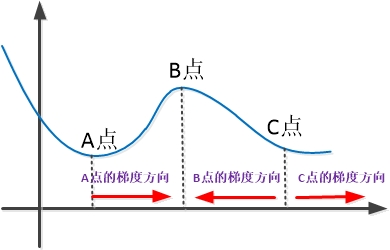其实一元函数肯定也有梯度，我们经常不提及的原因其实很简单：一元函数的梯度方向自变量轴（x）！而导数值的正负号决定了这个方向是正方向还是反方向。如图所示，A点右＂领域＂的导数为正值，则梯度的方向跟x轴正方向一致，梯度方向指向数值增大的方向；相反在B点右＂领域＂，导数为负值，则梯度的方向为x轴的负方向，梯度方向也是指向数值增大的方向。通过这个例子向多维函数推广，梯度从数值小指向数值大的物理意义也就容易理解了。而一元函数的大小自然也就是导数的绝对值。


展开全文函数
• 文章目录梯度和方向导数一、方向导数二、方向导数与偏导的关系三、梯度 一、方向导数 定义：若函数定义：若函数定义：若函数f(x,y,x)在点在点在点P(x,y,z)处沿方向处沿方向处沿方向l存在以下极限存在以下极限存在...
梯度和方向导数
文章目录梯度和方向导数一、方向导数二、方向导数与偏导的关系三、梯度
一、方向导数

$定义：若函数$f(x,y,x)$在点$P(x,y,z)$处沿方向$l$存在以下极限$
$\lim _{\rho \rightarrow 0} \frac{\Delta f}{\rho}=\lim _{\rho \rightarrow 0} \frac{f(x+\Delta x, y+\Delta y, z+\Delta z)-f(x, y, z)}{\rho}=\frac{\partial f}{\partial l} \left(\begin{array}{c}\rho=\sqrt{(\Delta x)^{2}+(\Delta y)^{2}+(\Delta z)^{2}}, \\ \Delta x=\rho \cos \alpha, \Delta y=\rho \cos \beta, \Delta z=\rho \cos \gamma\end{array}\right)$
$则称\frac{\partial f}{\partial l}为函数在点$P$处方向$l$的方向导数$
$定理：若函数f(x,y,z)在点P(x,y,z)处可微，则函数在该点沿任意方向l的方向导数存在，且有$
$\frac { \partial f } { \partial l } = \frac { \partial f } { \partial x } \cos \alpha + \frac { \partial f } { \partial y } \cos \beta + \frac { \partial f } { \partial z } \cos \gamma$
对于二元函数$f(x,y)$，在点$P(x,y)$处沿方向$l$的方向导数为
$\frac{\partial f}{\partial l}=f_{x}(x, y) \cos \alpha+f_{y}(x, y) \cos \beta$
二、方向导数与偏导的关系

$当l与x轴同向(\alpha=0,\beta=\frac{\pi}{2})时，有\frac{\partial f}{\partial l}=\frac{\partial f}{\partial x}$
$当l与x轴反向(\alpha=\pi,\beta=\frac{\pi}{2})时，有\frac{\partial f}{\partial l}=-\frac{\partial f}{\partial x}$
$\text{偏导存在}\Rightarrow \text{方向导数存在} \\ \text{方向导数存在}\nRightarrow \text{偏导存在}$

三、梯度

1.定义
$\vec{G}=\left(\frac{\partial f}{\partial x}, \frac{\partial f}{\partial y}, \frac{\partial f}{\partial z}\right)$
$向量\vec{G}称为函数f(p)在点处的梯度（gardient），记作\boldsymbol{gard}f(p)，或\nabla f(p)即$
$\operatorname{grad} f(P)=\nabla f(P)=\left(f_{x}(P), f_{y}(P), f_{z}(P)\right)$
$其中\nabla = \left(\frac{\partial}{\partial x}, \frac{\partial}{\partial y}, \frac{\partial}{\partial z}\right)称为向量微分算子或\boldsymbol{Nabla}算子.$
$同样可定义二元函数f(x,y)在点P(x,y)处的梯度$
$\operatorname{grad} f=\nabla f(x, y)=\left(f_{x}(x, y), f_{y}(x, y)\right)$
$说明：函数的方向导数为梯度在该方向上的投影：$
$\frac{\partial f}{\partial l}=\operatorname{grad} f\cdot \vec{l}^{0}$
2.梯度的几何意义
$对函数z=f(x,y)，曲线 \left\{\begin{array}{c}z=f(x, y) \\ z=c\end{array}\right.在xOy面上的投影L^*:f(x,y)=c称为函数f的\boldsymbol{等值线}或\boldsymbol{等高线}$
$设f_x，f_y不同时为零，则L_*上点P处的法向量为$
$\left.\left(f_{x}, f_{y}\right)\right|_{P}=\left.\operatorname{grad} f\right|_{P}=\left.\nabla f\right|_{P}$
$函数在一点的梯度垂直于该点等值线，指向函数增大的方向.$
$同样，f(x,y,z)=c称为u=f(x,y,z)的等值面(等量面).当其各偏导数不同时为零时，其上点P处的法向量\left.\operatorname{grad} f\right|_{P}=\left.\nabla f\right|_{P}.$
3.梯度的基本运算公式
$(1) \operatorname{grad} f=\vec{c}或\nabla c=\vec{0}(c为常数)$
$(2)\operatorname{grad}(cu)=c\operatorname{grad}u或\nabla(cu)=c\nabla(u)$
$(3)\operatorname{grad}(u\pm v)=\operatorname{grad}u \pm \operatorname{v}或\nabla(u \pm v)=\nabla u+\nabla v$
$(4)\operatorname{grad}(uv)=u\operatorname{grad}v+v\operatorname{u}或\nabla(uv)=u\nabla v+v\nabla u$
$(5)\operatorname{grad}(\frac{u}{v})=\frac{v\operatorname{grad}u-u\operatorname{grad}v}{v^2}或\nabla(\frac{u}{v})=\frac{v\nabla u-u\nabla v}{v^2}$
$(6)\operatorname{grad}f(u)=f^{\prime}\operatorname{grad}或\nabla f(u)=f^{\prime}(u)\nabla u$


展开全文• 最近做的东西需要用到牛顿法拟牛顿法，前两天自己在思考导数和梯度之间的关系的的时候，发现竟然不能清晰地表述出来。所以趁此机会再次复习一下。时间有限，这个也不是重点，所以主要对知乎上的一个相同问题中大家...
最近做的东西需要用到牛顿法和拟牛顿法，前两天自己在思考导数和梯度之间的关系的的时候，发现竟然不能清晰地表述出来。所以趁此机会再次复习一下。时间有限，这个也不是重点，所以主要对知乎上的一个相同问题中大家的解答，做一个总结，写的不够详细，希望不会误导读者，感兴趣的请移步原回答。
Ref：
如何直观形象的理解方向导数与梯度以及它们之间的关系？
导数
导数的概念和运用可以说是贯穿了我们自初中以来的所有数学知识。当自变量和因变量都是一维且定义域和值域都为实数域的情况下，自变量的导数主要用来描述自变量的某点关于因变量的瞬时变化率。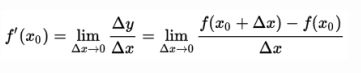从几何的角度来解释就是自变量该点关于函数的切线的斜率。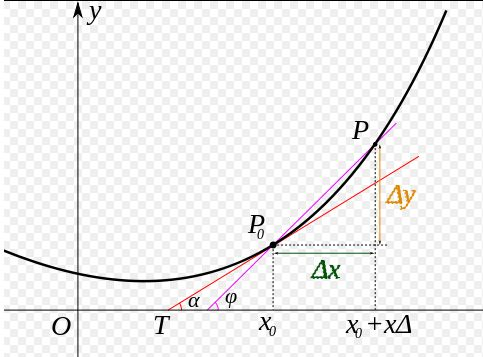注：有一个地方其实我当时迷了一下，当自变量只有一维的时候，该函数的梯度向量一定是和自变量的轴（也就是这里的x轴）平行的，方向和大小则由导数值决定
偏导数
而偏导数则是在因变量（函数值）为一元，自变量为多元的情况下，因变量关于各个自变量单独求导的导数。其中，各个自变量的偏导数分别表征了在某点它们关于函数值的瞬时变化率。从几何角度去解释的话，它们则分别代表了函数值在某点关于各个轴的切线的斜率。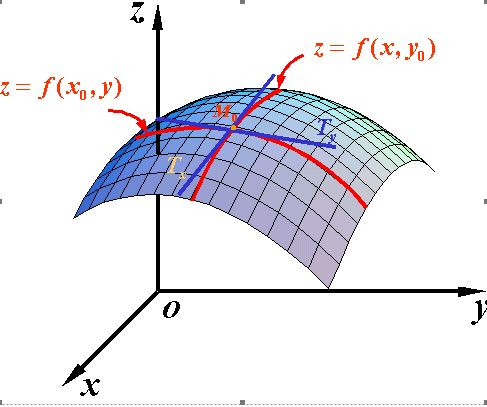方向导数与梯度
之前谈到的偏导数只能够帮助我们描述函数在各个轴上的变化率，若我们现在想要知道的是函数值关于某点在某个方向上的变化值怎么办。我们知道，当我们拥有两个正交的单位向量时，我们可以根据公式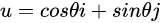来表征这两个向量构成的平面内的任一单位向量。同样的道理，当我们得到了函数关于各个自变量的偏导数的时候，我们就可以得到函数空间中任一方向导数的向量。以一个二元函数举例，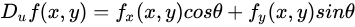经过观察我们不难发现，我们可以将上式写为两个向量点积的形式：
$D_uf(x,y) = dot((f_x(x,y),f_y(x,y)),(cos\theta,sin\theta))$
其中,$(f_x(x,y),f_y(x,y))$为函数的偏导数向量。向量点积，从几何的角度又可以被定义为两个响亮的投影，根据向量投影公式我们可以将上式变换为：
$D_uf(x,y) = |(f_x(x,y),f_y(x,y)||(cos\theta,sin\theta)|cos\alpha$
其中，$\alpha$表示两个向量之间的夹角，根据该定义我们可以得出当$\alpha$为0是该向量的模值最大。该模值即为偏导数向量的模值，该方向即为偏导数向量的方向，也就是说函数在该方向上拥有最大的瞬时变化率，这也和梯度的定义相契合。
一点个人的想法：
从上面的分析我们可以看出其实梯度就是各个偏导数向量的一个叠加。反过来讲，由于自变量之间的正交性，我们将各个偏导数向量叠加起来能够保证该向量仍然是整个函数变化最快的方向。


展开全文• 学习到机器学习线性回归逻辑回归时遇到了梯度下降算法，然后顺着扯出了一堆高数相关概念理论：导数、偏导数、全微分、方向导数梯度，重新回顾它们之间一些关系，从网上教材中摘录相关知识点。 通过函数...
学习到机器学习线性回归和逻辑回归时遇到了梯度下降算法，然后顺着扯出了一堆高数的相关概念理论：导数、偏导数、全微分、方向导数、梯度，重新回顾它们之间的一些关系，从网上和教材中摘录相关知识点。

通过函数的极限定义出导数(以一元函数为例)
函数f(x)在点x0可微的充分必要条件是函数f(x)在点x0处可导
扩展到多元函数时，衍生出偏导数

导数
定义：设函数$y=f(x)$在点$x_0$的某个领域内有定义，如果$\frac{Δy}{Δx}$在当$Δx$->0时极限存在，则称函数$y=f(x)$在$x_0$处可导，这个极限是函数$y=f(x)$在$x_0$处的导数
$f'(x_0)=\lim \limits_{Δx \to 0} \frac{Δy}{Δx}=\lim \limits_{Δx \to 0} \frac{f(x_0+Δx)-f(x_0)}{Δx}$
根据导数的定义，从某种意义上说导数的本质是一种极限

导数与导函数的关系是局部与整体的关系，导数通常是指一点，导函数则是指一个区间上的

在直线运动场景中，若x表示时刻，y表示距离，函数f表示时间与距离的关系$y=f(x)$,那么导数的含义就是在$x_0$时刻的瞬时速度
在直角坐标系中，$y=f(x)$表示一个曲线，导数的含义表示的是曲线在点$x_0$处的切线的斜率

微分
定义：设函数$y=f(x)$在某个领域内有定义，$x_0$及$x_0+Δx$在这区间内，如果增量
$Δy=f(x_0+x)-f(x_0)$
可表示为
$Δy=AΔx+o(Δx)$
其中A是不依赖$Δx$的常数，$o(Δx)$是指$Δx$趋于0时的高阶无穷小，那么称函数$y=f(x)$在点$x_0$是可微的，而$AΔx$叫做函数在点$x_0$相应于自变量增量$Δx$的微分，记作$\mathrm{d} y$，记作
$\mathrm{d}y=AΔx$

高阶无穷小的定义：如果$\lim \limits \frac{\beta}{\alpha}=0$，就说$\beta$是比$\alpha$高阶的无穷小，记作$\beta=o(\alpha)$

微分与导数的关系
上式$Δy=AΔx+o(Δx)$两边同时除以$Δx$得到
$\frac{Δy}{Δx}=A+\frac{o(Δx)}{Δx}$
当$Δx \to 0$时,上式左边就是导数的定义，而右边的$\frac{o(Δx)}{Δx}$因为是高阶无穷小，所以会趋向于0，得到以下等式
$A=\lim \limits_{Δx \to 0}\frac{Δy}{Δx}=f'(x_0)$
因此，如果函数$f(x)$在点$x_0$可微，则$f(x)$在点$x_0$也一定可导，且$A=f'(x_0)$，反之，如果$f(x)$在点$x_0$可导，存在下式
$\lim \limits_{Δx \to 0}\frac{Δy}{Δx}=f'(x_0)$
根据极限与无穷小的关系转化上式，当$Δx \to 0$时
$\frac{Δy}{Δx}=f'(x_0)+\alpha$
其中$\lim \limits_{Δx \to 0}a=0$，即$\lim \limits_{Δx \to 0}\frac{aΔx}{Δx}=0$,$aΔx=o(Δx)$，上式转化为下式(又回到了微分的定义)
$Δy=f'(x_0)Δx+o(Δx)$
因此，函数$f(x)$在点$x_0$可微的充分必要条件是函数$f(x)$在点$x_0$可导
$\mathrm{d}y=f'(x_0)Δx$
偏导数
一元函数的变化率是导数，多元函数的自变量有多个，当某个自变量x变化而其它自变量固定时，这时候对变化的自变量x进行求导，就称为多元函数对于x的偏导数。
定义：设函数$z=f(x,y)$在点$(x_0,y_0)$的某一领域内有定义，当$y$固定于$y_0$，而$x$在$x_0$处有增量$Δx$，相应的函数有增量
$f(x_0+Δx,y_0)-f(x_0,y_0)$
如果
$\lim \limits_{Δx \to 0}\frac{f(x_0+Δx,y_0)-f(x_0,y_0)}{Δx}$
存在，则称该极限为$z=f(x,y)$在点$(x_0,y_0)$处对$x$的偏导数
偏导数的几何意义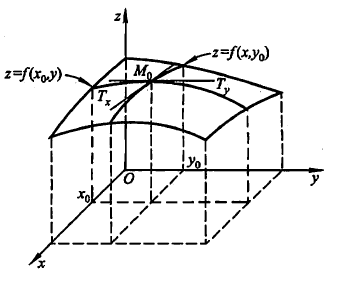偏导数$f_{x} (x_{0},y_{0} )$就是曲面被平面$y=y_{0}$所截得的曲线在点$M_{0}$处的切线$M_{0}T_{x}$对$x$轴的斜率
偏导数$f_{y} (x_{0},y_{0} )$就是曲面被平面$x=x_{0}$所截得的曲线在点$M_{0}$处的切线$M_{0}T_{y}$对$y$轴的斜率

很多时候要考虑多元函数沿任意方向的变化率，那么就引出了方向导数
全微分
参考上文微分的定义，与一元函数的情形一样，希望用自变量增量$Δx,Δy$来线性函数来代替函数的全增量$Δz$，从而减化计算
定义：设函数$z=f(x,y)$在点$(x,y)$的某领域内有定义如果函数在点$(x,y)$的全增量
$Δz=f(x+Δx,y+Δy)-f(x,y)$
可心表示为
$Δz=AΔx+BΔy+o(\rho)$
其中$A,B$不依赖于$Δx,Δy$，$\rho=\sqrt{(Δx)^2+(Δy)^2}$，则称函数$z=f(x,y)$在点$(x,y)$处可微分，而$AΔx+BΔy$称为函数在点$(x,y)$的全微分
$\mathrm{d}z=AΔx+BΔy$
可微分与偏导数关系
基于上述全微分定义成立，存在某一点$p'(x+Δx,y+Δy)$对于式子$Δz=AΔx+BΔy+o(\rho)$也成立，当$Δy=0$时
$f(Δx+x,y)-f(x,y)=AΔX+o(|Δx|)$
两边除以$Δx$并且令$Δx \to 0$取极限
$\lim \limits_{Δx \to 0}\frac{f(x+Δx,y)-f(x,y)}{Δx}=A$
这式子就是偏导数的定义形式啊，所以这说明了偏导数$f_x(x,y)$存在且等于$A$，同理也可证$f_y(x,y)=B$，由此推导出以下公式
$\mathrm{d}z=f_x(x,y)Δx+f_y(x,y)Δy$
各偏导数的存在只是全微分存在的必要条件而非充分条件，即由全微分可证各偏导数存在，反之则不行

如果函数的各个偏数在点$(x,y)$是连续的，则函数可微分

方向导数
定义导数、偏导数、方向导数都是说如果说某条件下极限存在，谨记导数的本质是极限及代表函数的变化率，偏导数反映的是函数沿坐标轴方向的变化率，有所限制，所以引入方向导数表示沿任意一方向的变化率
定义：设$l$是$xOy$平面以$P_0(x_0,y_0)$为始点的一条射线，$e_i=(cos\alpha,cos\beta)$是以射线同方向的单位向量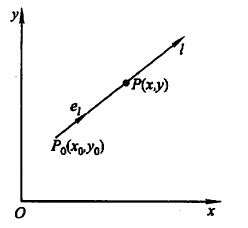射线$l$的参数方程为
$\begin{cases}x=x_0+tcos\alpha ，t\geq0\\ y=y_0+tcos\beta，t\geq0 \end{cases}$
如果函数增量$f(x_0+tcos\alpha,y_0+tcos\beta)-f(x_0,y_0)$与$P$到$P_0$的距离$|PP_0|=t$的比值，当点$P$沿着$l$趋于$P_0(即t \to 0^+)$时极限存在，则称此极限为函数在点$P_0$沿方向$l$的方向导数
$\frac{\partial f}{\partial l}|_{(x_0,y_0)}=\lim \limits_{t \to 0^+}\frac{f(x_0+tcos\alpha,y_0+tcos\beta)-f(x_0,y_0)}{t}$
方向导数与全微分的关系
由全微分的定义得到
$f(x_0+Δx,y_0+Δy)-f(x_0,y_0)=f_x(x_0,y_0)Δx+f_y(x_0,y_0)Δy+o(\sqrt{(Δx)^2+(Δy)^2})$
设点$(x_0+Δx,y_0+Δy)$在以$(x_0,y_0)$为起点的射线$l(cos\alpha,cos\beta是l的方向余弦)$上，则有$Δx=tcos\alpha$,$Δy=tcos\beta$,$\sqrt{(Δx)^2+(Δy)^2}=t$，所以
$\lim \limits_{t \to 0^+}\frac{f(x_0+Δx,y_0+Δy)-f(x_0,y_0)}{t}=f_x(x_0,y_0)cos\alpha+f_y(x_0,y_0)cos\beta$
上式左侧就是方向导数定义形式，极限存在即方向导数存在，且其值等于右式
由此得到定理，如果函数$f(x,y)$在点$P_0(x_0,y_0)$可微分，那么函数在该点沿任一方向$l$的方向导数存在
$\frac{\partial f}{\partial l}|_{(x_0,y_0)}=f_x(x_0,y_0)cos\alpha+f_y(x_0,y_0)cos\beta$
梯度
在平面上确定某一点可能存在无数个方向导数，我们怎样找到其中一个方向导数来描述函数最大变化率？
定义：在二元函数的情形，	设函数$f(x,y)$在平面区域D内具有一阶连续偏导数，对于每一点$P_0(x_0,y_0)\in D$，都可以给出一个向量
$f_x(x_0,y_0)i+f_y(x_0,y_0)j \quad 或用坐标表示 \quad (f_x(x_0,y_0),f_y(x_0,y_0))$
其中$i,j$为$x,y$轴的方向向量，上述微量称为函数$f(x,y)$在点$P_0(x_0,y_0)$的梯度记作
$gradf(x_0,y_0)=f_x(x_0,y_0)i+f_y(x_0,y_0)j$
由定义看到，梯度的方向是确定的，如果点$P$的坐标确定，那么梯度也大小也确定
如果函数$f(x,y)$在点$P_0(x_0,y_0)$可微分，$e_l=(cos\alpha,cos\beta)$是方向$l$的方向向量(方向未确定)
$\frac{\partial f}{\partial l}|_{(x_0,y_0)}=f_x(x_0,y_0)cos\alpha+f_y(x_0,y_0)cos\beta=grad\ f(x_0,y_0).e_l=|grad\ f(x_0,y_0)|cos\theta$
其中$\theta$为向量${grad\ f(x_0,y_0)}$与向量$e_l$的夹角，当$\theta=0$时，即方向$e_l$与梯度${grad\ f(x_0,y_0)}$的方向时，函数$f(x,y)$增加最快，函数在这个方向的方向导数达到最大值，这个值就是梯度${grad\ f(x_0,y_0)}$的模，即
$\frac{\partial f}{\partial l}|_{(x_0,y_0)}=|grad \ f(x_0,y_0)|$
所以可以用沿梯度方向的方向导数来描述是函数最大变化率，即梯度方向是函数变化率最大的方向，在梯度定义的时候就已经赋予了它这个特性。


展开全文高等数学 机器学习
• 偏导数、方向导数梯度之间微妙的关系 偏导数：函数某点沿一个某一个维度的变化率，是一个数值 方向导数：函数某点沿一个某一个方向的变化率，是一个数值 梯度：函数某点变化率最大的方向，是一个向量 可以看出偏...
• 突然想到权重更新首先是通过输出函数对权重求偏导，之后在原有权重值得情况下进行更新（减去或者加上偏导值），那难道求到偏导就是梯度嘛，这样岂不是梯度和偏导是同样含义，梯度和偏导之间又有什么关系呢？...深度学习 神经网络
• 我们先来玩一个游戏，假如你在一座山上，蒙着眼睛，但是你必须到达山谷中最低点湖泊，你该怎么办？ 梯度可以帮助你完成这...梯度和方向导数紧密相关，让我们从方向导数开始。 1 方向导数 顾名思义，方向导数...
• 学习到机器学习线性回归逻辑回归时遇到了梯度下降算法，然后顺着扯出了一堆高数相关概念理论：导数、偏导数、全微分、方向导数梯度，重新回顾它们之间一些关系，从网上教材中摘录相关知识点。 通过函数...偏导数
• 梯度和方向导数 在一个二维平面中，如果温度T和平面中某一个点位置的关系是： T=f(x,y) 我们都知道x的偏导数是该函数在x轴上的变化率。y的偏导数是该函数在y轴上的变化率。那如果，我想知道，这个函数在y=x这...数学建模
• 这里只讨论梯度下降法和梯度之间的关系，先让我们了解一下导数、偏导数、方向导数和梯度的概念。导数：定义就不讲了，含义：一元函数在某一点的导数描述了这个函数在这一点附近的变化率。几何意义：一元函数曲线在...偏导数
• 教学目的：掌握方向导数的定义求法；掌握梯度的定义、求法及其与等高线的关系. 教学重点：方向导数与梯度的求法. 教学难点：方向角的确定. 教学内容： 一、方向导数 现在我们来讨论函数在一点沿某一方向的...
• 3.微分与导数的关系 4.偏导 类比于一元函数，也想研究函数的变化率问题，在日常生活中，我们经常遇到这样的问题，一个值许多元素相关，我们习惯只改变一个变量值，其它变量值固定，看变化的情况。 这
• 学习到机器学习线性回归逻辑回归时遇到了梯度下降算法，然后顺着扯出了一堆高数相关概念理论：导数、偏导数、全微分、方向导数梯度，重新回顾它们之间一些关系，从网上教材中摘录相关知识点。 这段是我...
• 之前一直错误的认为了切向量就是梯度，由于最近在看拉格朗日的方法，正好遇到这个问题，结果搞清楚了切向量和梯度的关系。 简单的来说切向量和梯度都是求导数，但是要看的是对谁求导数。举个例子： y=x^2吧，...
• 文章目录梯度导数的关系梯度下降算法梯度方向是上升方向一阶泰勒展开式与负梯度 梯度导数的关系 梯度方向指向数值增长最快的方向，大小为变化率。通过这个性质也说明梯度是有方向大小的矢量。通过梯度的定义...
• 以下，我们在图像处理中默认梯度，导数，差分是一个概念。 我们前面博客中关于自动对焦，使用了梯度和=|dx|+|dy|的方法解决。 我们上一节证明了积分和...这就是积分和与梯度和以及盒子（box）滤波器的关系。 所以，
• 梯度下降（Gradient Descent）小结 - 刘建平Pinard - 博客园: ...梯度和导数的关系 梯度下降： 1.梯度是一个向量 2.梯度的大小就是 该点最大的倒数 某一点会有各种斜率值（不同方向），其中沿梯度方...
• 1.梯度和方向导数有很大关系。 2.梯度定义：梯度本意是一个向量（矢量），表示某一函数在该点处方向导数沿着该方向取得最大值，即函数在该点处沿着该方向（此梯度方向）变化最快，变化率最大（为该梯度模...
• 由各向异性扩散原理与边缘...基于查找方法通过寻找图像一阶导数最大最小值来检测边界，通常是将边界定位在梯度最大方向。基于零穿越方法通过寻找图像二阶导数零穿越来寻找边界，通常是Laplacian过零点或
• 通过计算梯度扩张系统的输出函数沿着输入向量场系统向量场的李导数，讨论仿射非线性控制系统的梯度扩张系统的能达性分布，研究了非线性控制系统它的梯度扩张系统的能达性之间的关系，证明了如果梯度扩张系统是...
• ## 数学：梯度的理解

千次阅读 2019-04-16 11:21:45
方向导数的解释 函数 z=f(x,y) 表示空间曲面 S，则点 P(x0, y0,z0) 在 S 上，过...方向导数和梯度的关系 当 u 与 ▽f 同方向时, 函数 f 增加最快, 类似, 反方向减少最快.。而正交于梯度的方向 u 是 f 变化率为 0 ...
• 对应深度学习入门104页 梯度下降法是什么 证明 什么是方向余弦 什么是方向导数 就是某个点向着某个方向前进一点点所...方向导数和梯度有什么关系 方向导数什么时候有最大值 即某个点的梯度方向有方向导数max ...
• 如果不理解梯度和方向导数的关系：可以看看：如何直观形象的理解方向导数与梯度以及它们之间的关系 梯度下降法还可能看看：梯度下降（Gradient Descent）小结 反向传播法：依次看以下的文章： 小白也能看懂的BP......